# The Substitution Method

[Page D-2 ( continued )]

The least complex method for solving nonlinear programming problems is referred to as substitution . This method is restricted to models that contain only equality constraints, and typically only one of these. The method involves solving the constraint equation for one variable in terms of another. This new expression is then substituted into the objective function, effectively eliminating the constraint. In other words, a constrained optimization model is transformed into an unconstrained model.

In the substitution method the constraint equation is solved for one variable in terms of another and then substituted into the objective function .

For an example of the substitution method we will use a profit analysis model. This is a nonlinear model that we introduced in chapter 10 of the text. The demand function is a constraint. The nonlinear programming model is formulated as

maximize Z = vp c f vc v

subject to

v = 1,500 24.6 p

The objective function in this model is nonlinear, because both v (volume) and p (price) are variables and multiplying them (i.e., vp ) creates a curvilinear relationship.

The constraint has already been solved for one variable ( v ) in terms of another ( p ); thus, we can substitute this expression directly into the objective function. This results in the following unconstrained function.

Z = 1,500 p 24.6 p 2 + c f 1,500 c v + 24.6 pc v

By substituting the constant values for c f (\$10,000) and c v (\$8), we obtain

Z = 1,696.8 p 24.6 p 2 22,000

Next, we solve this problem by differentiating the function Z and setting it equal to zero.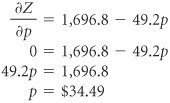[Page D-3]

We will present another example as a further illustration of a nonlinear programming problem and the substitution method. In this example the Beaver Creek Pottery Company produces bowls ( x 1 ) and mugs ( x 2 ). We will assume that the profit contribution for each product declines as the quantity of each item produced increases . Thus, for bowls the per unit profit contribution is now expressed according to the relationship

\$4 0.1 x 1

For mugs, the profit contribution per unit is

\$5 0.2 x 2

(These relationships express the fact that production costs for each product increase as the number of units sold increases.)

These profit relationships are on a per unit basis. Thus, the total profit contribution from each product is determined by multiplying these relationships by the number of units produced. For bowls, the profit contribution is

(4 0.1 x 1 ) x 1

or

4 x 1 0.1 x 2 1

For mugs, the profit contribution is

(5 0.2 x 2 ) x 2

or

5 x 2 0.2 x 2 2

Total profit is the sum of these two terms.

Z = \$4 x 1 0.1 x 2 1 + 5 x 2 0.2 x 2 2

In this model, we will consider only a labor constraint, and we will treat it as an equality rather than an inequality.

x 1 + 2 x 2 = 40 hr

The complete nonlinear programming model is as follows .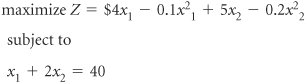The first step in the substitution method is to solve the constraint equation for one variable in terms of another. We will arbitrarily decide to solve for x 1 as follows.

x 1 = 40 2 x 2

Now wherever x 1 appears in the nonlinear objective function, we will substitute the expression 40 2 x 2 .

 Z = 4(40 2 x 2 ) 0.1(40 2 x 2 ) 2 + 5 x 2 0.2 x 2 2 = 160 8 x 2 0.1(1,600 160 x 2 + 4 x 2 2 ) + 5 x 2 0.2 x 2 2 = 160 8 x 2 160 + 16 x 2 0.4 x 2 2 + 5 x 2 0.2 x 2 2 = 13 x 2 0.6 x 2 2

[Page D-4]

This is an unconstrained optimization function, and we can solve it by differentiating it and setting it equal to zero.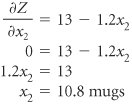To determine x 1 , we can substitute x 2 into the constraint equation.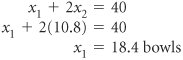Substituting the values of x 1 and x 2 into the original objective function gives the total profit, as follows.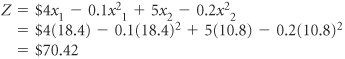Both of the examples presented in this section for solving nonlinear programming problems exhibit the limitations of this approach. The objective functions were not very complex (i.e., the highest order of a variable was a power of two in the second example), there were only two variables, and the single constraint in each example was an equation. This method becomes very difficult if the constraint becomes complex. An alternative solution approach that is not quite as restricted is the method of Lagrange multipliers.Introduction to Management Science (10th Edition)
ISBN: 0136064361
EAN: 2147483647
Year: 2006
Pages: 358

Similar book on Amazon

flylib.com © 2008-2017.
If you may any questions please contact us: flylib@qtcs.net